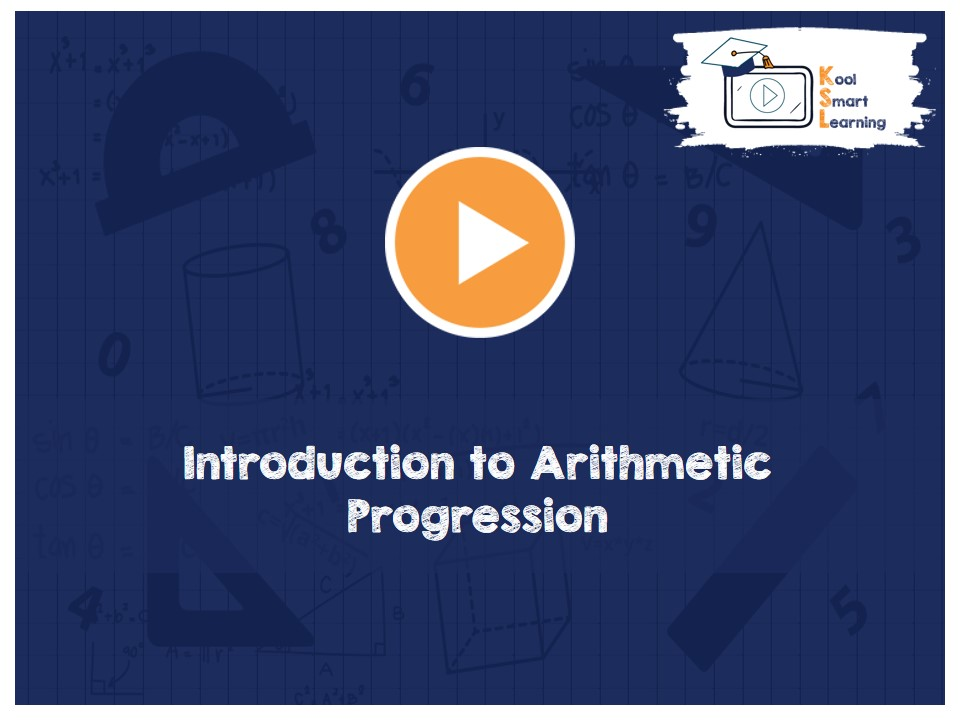At KoolSmartLearning, we intend to harness the power of online education to make learning easy.## Introduction to Arithmetic Progression

/  Introduction to Arithmetic Progression## Introduction to Arithmetic Progression

The video explains what are sequences, progressions in general and Arithmetic Progression(A.P) in specific. It also talks about the general term of an A.P, finite & infinite A.P. The video includes finding the nth term of an A.P from the beginning or from the end with the derivation of the formula. The selection of terms while solving A.P. related problems is also explained in the video.

More Related Videos